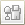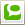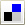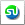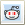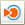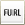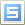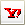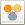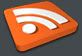Delivered by FeedBurner## MAKE IMAGES ON INTERNET DANCE

Labels:
BOOKMARK

MAKE IMAGES ON INTERNET DANCE.
A Javascript trick that makes all the images on the site your on float and dance around. :]

You can do this to ANY site with images

OPEN UR BROWSER
OPEN AYN SITE THAT CONTAINS IMAGES
Go to the address bar on top where site name is there eg.www.trickscafe.blogspot.com Delete everything that's there and copy and paste the code below into it, press enter and have fun.

javascript:R=0; x1=.1; y1=.05; x2=.25; y2=.24; x3=1.6; y3=.24; x4=300; y4=200; x5=300; y5=200; DI=document.getElementsByTagName("img"); DIL=DI.length; function A(){for(i=0; i-DIL; i++){DIS=DI[ i ].style; DIS.position='absolute'; DIS.left=(Math.sin(R*x1+i*x2+x3)*x4+x5)+ "px"; DIS.top=(Math.cos(R*y1+i*y2+y3)*y4+y5)+" px"}R++}setInterval('A()',5); void(0);

BOOKMARK